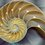This is the first post of a series devoted to Number theory. I decided to start it off with a well-known fact about the primes in the form $4k+3$.This fact can be extremely useful in solving problems.

Lemma: If $x^{2}+y^{2}$ is divisible by a prime in the form $p=4k+3$, then p$\mid$x and p$\mid$y.

Proof:First let's note that if one of the numbers x and y is divisible by p, then so is the other.Let's prove the lemma by contradiction (reductio ad absurdum). So let $x^2+y^2\equiv0\pmod{p}$ and both x and y are coprime to p.Then $x^2\equiv-y^2\pmod{p}\Rightarrow x^{p-1}\equiv-y^{p-1}\pmod{p}$.We have just risen the both sides to the power of $\frac{p-1}{2}=2k+1$.But by Fermat's little theorem (since x and y are coprime to p) we get that $1\equiv-1\pmod{p}$, which is impossible $\Rightarrow$p divides at least one of the numbers x and y , but as we said before, that means that p divides both of them!

So from this lemma we can see that if a number can be represented as the sum of two squares of natural numbers, then in its prime factorization primes of the form 4k+3 are included in an even power. So if $n = a^2 + b^2$, then $\upsilon_p(n)=2r$ for some natural numbers a, b and r.Now for a little bit of exercise I will post a problem for you to try yourself.

Problem:Show that $4xy -x-y=z^2$ has no solutions in natural numbers.

Hint:Try to factorize.Note by Bogdan Simeonov
6 years, 9 months ago

This discussion board is a place to discuss our Daily Challenges and the math and science related to those challenges. Explanations are more than just a solution — they should explain the steps and thinking strategies that you used to obtain the solution. Comments should further the discussion of math and science.

When posting on Brilliant:

• Use the emojis to react to an explanation, whether you're congratulating a job well done , or just really confused .
• Ask specific questions about the challenge or the steps in somebody's explanation. Well-posed questions can add a lot to the discussion, but posting "I don't understand!" doesn't help anyone.
• Try to contribute something new to the discussion, whether it is an extension, generalization or other idea related to the challenge.

MarkdownAppears as
*italics* or _italics_ italics
**bold** or __bold__ bold
- bulleted- list
• bulleted
• list
1. numbered2. list
1. numbered
2. list
Note: you must add a full line of space before and after lists for them to show up correctly
paragraph 1paragraph 2

paragraph 1

paragraph 2

[example link](https://brilliant.org)example link
> This is a quote
This is a quote
    # I indented these lines
# 4 spaces, and now they show
# up as a code block.

print "hello world"
# I indented these lines
# 4 spaces, and now they show
# up as a code block.

print "hello world"
MathAppears as
Remember to wrap math in $$ ... $$ or $ ... $ to ensure proper formatting.
2 \times 3 $2 \times 3$
2^{34} $2^{34}$
a_{i-1} $a_{i-1}$
\frac{2}{3} $\frac{2}{3}$
\sqrt{2} $\sqrt{2}$
\sum_{i=1}^3 $\sum_{i=1}^3$
\sin \theta $\sin \theta$
\boxed{123} $\boxed{123}$

Sort by:

Multiplying by 4 and adding 1 yields:

$(4x-1)(4y-1)=4z^2+1$

Now let $p=4k+3$ be a prime for some integer $k$. We have that $p \mid LHS$ so $p\mid RHS$. By the theorem in the post, we must then have $p \mid 1$. But $p>1$, so there are no solutions.

- 6 years, 9 months ago

Since both the factors on the LHS are of the form 4k+3 it must have a prime fsctor of that form and hence your proof follows right?

- 6 years, 9 months ago

Yep, that is how it's supposed to be.

- 6 years, 9 months ago

I am sorry if the title was misleading!Anyways, if you found this post helpful in any way, make sure to keep track of my future posts!Also, make sure to post your solutions to the problem at the end of the post!

- 6 years, 9 months ago

Good, but a better title might have been "primes and sum of 2 squares" or something like that, just a suggestion.

A good exercise is also by this lemma, and Fermat's theorem on sum of two squares, characterize all integers that can be represented as sum of two squares.

- 6 years, 9 months ago

Maybe I will do that...but I think it's better by starting with something simpler.

- 6 years, 9 months ago

Yea it is a nice result by this. That Fermat theorem might be pretty hard to prove...But maybe you can consider.

- 6 years, 9 months ago

Fermat's result is very difficult to prove - it uses quadratic residues, order, and Gaussian integers, pulling together many of the important facts of elementary number theory.

- 6 years, 9 months ago

It is hard, but there are several proofs...Check out Euler's proof using infinite descent,which uses pretty elementary number theory. I also saw a proof that uses Thue's theorem.

- 6 years, 9 months ago

The infinite descent proof was about Fermat's Last Theorem for the cubes, wasn't it?Or is there such a proof for the squares theorem?

- 6 years, 9 months ago

There is an infinite descent proof for this too.

- 6 years, 9 months ago